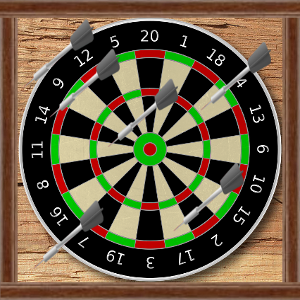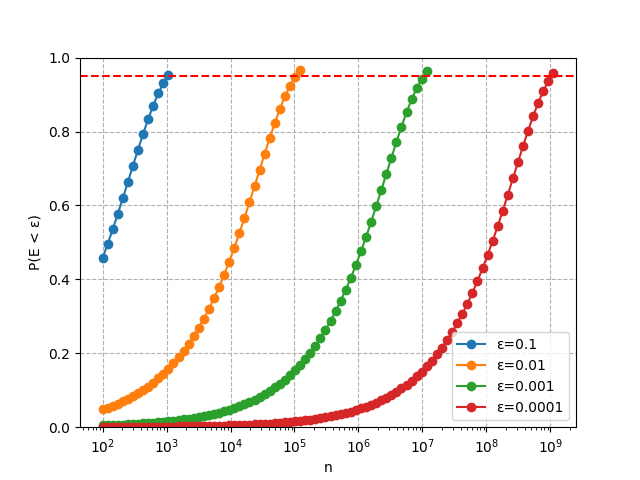## The Darts Player Who Estimated π

### A story of darts and Monte Carlo methods.

A computational maths student walked into a bar. She had read a footnote somewhere which claimed that it was possible to estimate π, the most famous constant in mathematics, using nothing but darts. And she was there to test this idea out.

A dartboard in the corner caught her eye. She walked over, picked up a few darts, and chucked them at the board. She wasn't a talented darts player, and the darts distributed themselves without a noticeable pattern. Almost... at random.Here was the gist of her experiment. If she could estimate the area of the dartboard, then she'd get an estimate of π for free, because the area of a circle is $A=\pi r^2$, which rearranges to $\pi = A/r^2$. She could figure out the radius easily enough using measuring tape, but how to estimate the area? By throwing darts, of course.

Given how bad she was at darts, each dart she threw would hit a random point within the dartboard's square wooden frame. A fraction of them, $f$, would land on the dartboard itself. This $f$ would be an estimate of how much of the frame was covered by the dartboard. The frame, being a square, had an area of about $2r\times 2r=4r^2$, since its sides were about the same as the diameter of the dartboard. And so the area of the dartboard could be estimated by $A=4fr^2$.

Just as she was about to run off in search of measuring tape for the sides of the frame, she realised something. Plugging $A$ back into the formula for π gave

\begin{aligned} \pi&=(4fr^2)/(r^2) \\ &= 4f. \end{aligned}

She wouldn't need measuring tape after all. $f$ could be estimated using nothing but darts!

She began to throw, keeping a diligent count of where the darts landed. 10 darts, 20 darts, 50 darts. She stopped after 100 throws, when her arm got sore. 81 darts had hit the dartboard, which gave an estimate of $\pi = 4(81/100) = 3.24$. It was in the same ballpark as π, but it seemed like she would need to throw a lot more darts to improve her estimate. And her arm was already dead from throwing. If only there were a way to automate this tedious process.

But of course! That's what she was studying computational maths for. She raced home to her computer and wrote the following code to simulate the dart throws. She occupied an alternate universe where Common Lisp was the most popular programming language, so that's the programming language she used. Her code took a single parameter, n, and simulated n dart throws. The output was an estimate of π.

(defun estimate-pi (n)
(let ((in-circle-count
(loop repeat n
sum (if (apply #'is-in-circle-p (random-xy)) 1 0))))
(* 4 (/ in-circle-count n))))

(defun random-xy ()
(loop repeat 2
collect (- 1/2 (random (* 2.0 1/2)))))

(defun is-in-circle-p (x y)
(<= (sqrt (+ (square x) (square y))) 1/2))

(defun square (x)
(* x x))


She simulated 1000 throws through her Common Lisp interpreter.

> (estimate-pi 1000)
3.104


Wonderful. She would merely have to increase the number of throws and her estimate would get better and better. Soon she'd have the most accurate estimate of π in the world!

> (estimate-pi 10000)
3.1356
> (estimate-pi 100000)
3.14144
> (estimate-pi 1000000)
3.142252
> (estimate-pi 10000000)
3.1426332


Except, after simulating 10 million throws, her approximation was still only accurate to 2 decimal places! Her computer's fan was getting noisy, and she didn't want to push it over the edge. Instead, she sat back and wondered how many darts she would have to throw in order to achieve an accuracy of, say, 4 decimal places. Luckily, her course included a module on probability, so she would probably be able to figure it out.

She knew that she could model the number of darts to hit the dartboard as a binomial random variable, $X$ -- a random variable being a variable that takes on each of its possible values with a certain probability. In this case, if she threw $n$ darts, then $X$ could take on any value from $0$ to $n$. Her estimate $Y$ could be modelled as another random variable, $Y=4X/n$. The error in her estimate could be modelled by its distance from the actual value of π, so $E=\vert Y-\pi\vert$.

After all that, she could estimate the probability of the error being less than some small value, $\epsilon$.

\begin{aligned} P(E < \epsilon) &= 1-2P(Y-\pi < -\epsilon) \\ &= 1-2P(4X/n < \pi-\epsilon) \\ &= 1-2P(X < n(\pi-\epsilon)/4). \end{aligned}

How could she calculate $P(X < n(\pi-\epsilon))$? The probability of a binomial random variable being less than some number $k$ is

$P(X < k) = \sum^{k-1}_{i=0} {n \choose i}p^i(1-p)^{n-i},$

where $p$, in the case of the darts experiment, would be the probability of an individual dart hitting the dartboard, $p=\pi/4$. It would take too long to calculate this sum as $k$ grew into the billions. Thankfully, she knew that binomial random variables can be approximated using a normal distribution with mean $\mu=np$ and standard deviation $\sigma=\sqrt{np(1-p)}$.

That's how she produced the following graph, which indicated that an accuracy beyond 3 or 4 decimal places was a hopeless cause. Back when she threw 100 darts, there had been about a 50% chance of her estimate being accurate to 1 decimal place. For a 95% chance of 4 decimal places of accuracy, she would need to throw over 1 billion darts.A few days later, the student was explaining her dart experiment to a friend.

"It seems you've squeezed as much as you can out of π," said the friend. "What will your next project be?"

"Well... have you heard of e?"

### Appendix A: background

To see the darts experiment in action, watch this video (Physics Girl, "Calculating Pi with Darts").

This story was inspired by exercise 3.5 of the computer science textbook, Structure and Interpretation of Computer Programs. It asks you to estimate π using a Monte Carlo algorithm. In other words, by simulating a bunch of dart throws.

### Appendix B: the code, explained

If you're familiar with programming but not the Common Lisp programming language, this appendix will guide you through the full program for estimating π.

First, we define a simple utility function for squaring a number. defun is the keyword for defining a function, square is the name of our function, and x is the function parameter.

(defun square (x)
(* x x))


In the function body, you'll notice that there's no return statement. That's because the return value of a function is the value of the final expression in the function body, which in this case is (* x x). You'll also notice that the multiplication operator, *, comes before its operands. It's treated just like a function, so in the same way that we'd write (square 5) with our new function to square the number 5, we write (* 1 2) to multiply the numbers 1 & 2.

Now we define a function to generate random x & y coordinates within the square, returning a list (x y). The function has no parameters; we call it like (random-xy). In the function body, we repeat our procedure to generate a random coordinate, once for x and once for y, and collect the results in a list. The circle is centered at (0,0), so the random values we generate should be between -0.5 and +0.5.

(defun random-xy ()
(loop repeat 2
collect (- 1/2 (random (* 2.0 1/2)))))


We need one last helper function. Coordinates x & y lie within a circle if they satisfy:

$\sqrt{x^2 + y^2} \leq r.$

Here it is in code. We follow the convention that a function name should end in "-p" if it returns a true or false value, p being short for predicate.

(defun is-in-circle-p (x y)
(<= (sqrt (+ (square x) (square y))) 1/2))


Finally, the meat of the code! Here's the function to simulate a given number of dart throws and return an estimate of π based on the results, just like in the story.

(defun estimate-pi (n)
(let ((in-circle-count
(loop repeat n
sum (if (apply #'is-in-circle-p (random-xy)) 1 0))))
(* 4 (/ in-circle-count n))))


We let a local variable, in-circle-count, equal the number of darts that land on the dartboard. This number is calculated by looping n times and summing 1 if a dart throw lands on the board, or 0 if it doesn't. apply takes each pair of coordinates that we generate, (x y), and passes it to is-in-circle-p. Finally, within the body of the let, and using the value of in-circle-count, we calculate our estimate.

If you want to learn more about Lisp, I recommend taking a look at The Little Schemer.

I'd be happy to hear from you at galligankevinp@gmail.com.